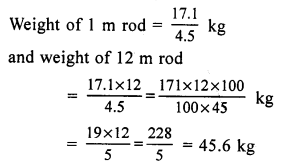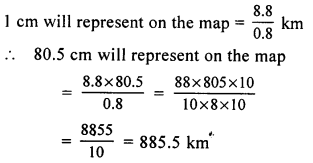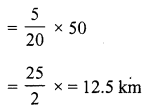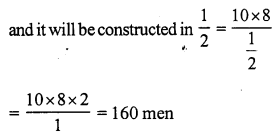# RS Aggarwal Class 7 Solutions Chapter 9 Unitary Method Ex 9C

## RS Aggarwal Class 7 Solutions Chapter 9 Unitary Method Ex 9C

These Solutions are part of RS Aggarwal Solutions Class 7. Here we have given RS Aggarwal Solutions Class 7 Chapter 9 Unitary Method Ex 9C.

Other Exercises

Objective Questions.
Marks (✓) against the correct answer in each of the following :
Question 1.
Solution:
(c)
Weight of 4.5 m rod = 17.1 kgQuestion 2.
Solution:
(d) None of these 0.8 cm represent the map = 8.8 kmQuestion 3.
Solution:
(c) In 20 minutes, Raghu covers = 5 km
in 1 minutes, he will cover = $$\frac { 5 }{ 20 }$$ km
and in 50 minutes, he will coverQuestion 4.
Solution:
(d)
No. of men in the beginning = 500
More men arrived = 300
No. of total men = 500 + 300 = 800
For 500 men, provision are for = 24 days
For 1 man, provision will be = 24 x 500 days (less men, more days)
and for 800 men, provision will be = $$\frac { 24 }{ 800 }$$ x 500 days
(more men less days)
= 15 days

Question 5.
Solution:
(b) Total cistern = 1
Filled in 1 minute = $$\frac { 4 }{ 5 }$$
Unfilled = 1 – $$\frac { 4 }{ 5 }$$ = $$\frac { 1 }{ 5 }$$
$$\frac { 4 }{ 5 }$$ of cistern is filled in = 1 minutes = 60 seconds
1 full cistern can be filled in = $$\frac { 60 x 5 }{ 4 }$$ = 75 seconds
More time = 75 – 60 = 15 seconds

Question 6.
Solution:
(a)
15 buffaloes can eat as much as = 21 cows
1 buffalo will eat as much as = $$\frac { 21 }{ 15 }$$ cows
35 buffaloes will eat as much as
= $$\frac { 21 x 35 }{ 15 }$$ cm = 49 cows

Question 7.
Solution:
(b) 4 m long shadow is of a tree of height = 6 m
1 m long shadow of flagpole will of height = $$\frac { 6 }{ 4 }$$ m
50 m long shadow, the height of pole 6 will be = $$\frac { 6 }{ 4 }$$ x 50 = 75 m

Question 8.
Solution:
(b) 8 men can finish the work in = 40 days
1 man will finish it in=40 x 8 days (less men, more days)
8 + 2 = 10 men will finish it in = $$\frac { 40 x 8 }{ 10 }$$ days
(more men, less days)
= 32 days

Question 9.
Solution:
(b)
16 men can reap a field in = 30 days
1 man will reap the field in = 30 x 16 days
and 20 men will reap the field in = $$\frac { 30 x 16 }{ 20 }$$ = 24 days

Question 10.
Solution:
(c) 10 pipe can fill tank in = 24 minutes
1 pipe will fill it in = 24 x 10 minutes (less pipe, more time)
and 10 – 2 = 8 pipes will fill the tank in
= $$\frac { 24 x 10 }{ 8 }$$ = 30 minutes

Question 11.
Solution:
(d) 6 dozen or 6 x 12 = 72 eggs
Cost of 72 eggs is = Rs. 108
Cost of 1 egg will be = Rs. $$\frac { 108 }{ 72 }$$
and cost of 132 eggs will be 108
= Rs. $$\frac { 108 }{ 72 }$$ x 132 = Rs. 198

Question 12.
Solution:
(b) 12 workers take to complete the work = 4 hrs.
1 worker will take = 4 x 12 hrs. (less worker, more time)
15 workers will take = $$\frac { 4 x 12 }{ 15 }$$ hrs. (more workers, less time)
= $$\frac { 16 }{ 5 }$$ hr. = 3 hrs. 12 min

Question 13.
Solution:
(a) 27 days – 3 days = 24 days
Men = 500 + 300 = 800
For 500 men, provision is sufficient = 24 days
For 1 man, provision will be = 24 x 500 (less man, more days)
and for 500 + 300 = 800 men provision
will be sufficient = $$\frac { 24 x 500 }{ 800 }$$ = 15 days
(more men, less days)

Question 14.
Solution:
(c) No. of rounds of rope = 140
Radius of base of cylinder = 14 cm
Radius of second cylinder of cylinder = 20 cm
If radius is 14 cm, then rounds of rope are = 140
If radius is 1 cm, then round = 140 x 14 (less radius more rounds)
and if radius is 20 cm, then rounds will
be = $$\frac { 140 x 14 }{ 20 }$$ = 98 (more radius less rounds)

Question 15.
Solution:
(d) A worker makes toy in $$\frac { 2 }{ 3 }$$ hr= 1
He will make toys in 1 hr = 1 x $$\frac { 3 }{ 2 }$$
and will make toys in $$\frac { 22 }{ 3 }$$ hrs. = 1 x $$\frac { 3 }{ 2 }$$ x $$\frac { 22 }{ 3 }$$
= 11 (more time more toys)

Question 16.
Solution:
(d) A wall is constructed in 8 days by = 10 men
It will be constructed in 1 day by = 10 x 8 men (less time, more men)
10 x 8More men required = 160 – 10 = 150

Hope given RS Aggarwal Solutions Class 7 Chapter 9 Unitary Method Ex 9C are helpful to complete your math homework.

If you have any doubts, please comment below. Learn Insta try to provide online math tutoring for you.